# Visualization In Python IV : Venn, Density, Violin Graphs

Hey good to see you back, I hope you are following the previous articles if not you should check them out, its not necessary to know those topics for this tutorial but it will surely increase your knowledge.

Well know lets continue from where we left off.

# 1. Venn Diagram

Venn diagrams, also known as set diagrams, show all possible logical relations between a finite collection of different sets. Each set is represented by a circle. The circle size illustrates the importance of a group. The size of overlap represents the intersection between multiple groups.

To show overlaps for different sets.

From the preceding diagram, we can note that there are eight students in just group A, four students in just group B, and one student in both groups.

Design Practice

• It is not recommended to use Venn diagrams if you have more than three groups. It would become difficult to understand.

Moving on from composition plots, we will cover distribution plots in the following section.

# Distribution Plots

Distribution plots give a deep insight into how your data is distributed. For a single variable, a histogram is effective. For multiple variables, you can either use a box plot or a violin plot. The violin plot visualizes the densities of your variables, whereas the box plot just visualizes the median, the interquartile range, and the range for each variable.

# 1. Histogram

A histogram visualizes the distribution of a single numerical variable. Each bar represents the frequency for a certain interval. Histograms help get an estimate of statistical measures. You see where values are concentrated, and you can easily detect outliers. You can either plot a histogram with absolute frequency values or, alternatively, normalize your histogram. If you want to compare distributions of multiple variables, you can use different colors for the bars.

Get insights into the underlying distribution for a dataset.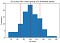distribution of the Intelligence Quotient ( IQ) for a test group. The dashed lines represent the standard deviation each side of the mean (the solid line)

Design Practice

Try different numbers of bins (data intervals), since the shape of the histogram can vary significantly.

# 2. Density Plot

A density plot shows the distribution of a numerical variable. It is a variation of a histogram that uses kernel smoothing, allowing for smoother distributions. One advantage these have over histograms is that density plots are better at determining the distribution shape since the distribution shape for histograms heavily depends on the number of bins (data intervals).

To compare the distribution of several variables by plotting the density on the same axis and using different colors.

Design Practice

Use contrasting colors to plot the density of multiple variables.

# 3. Box Plot

The box plot shows multiple statistical measurements. The box extends from the lower to the upper quartile values of the data, thus allowing us to visualize the interquartile range (IQR). The horizontal line within the box denotes the median. The parallel extending lines from the boxes are called whiskers; they indicate the variability outside the lower and upper quartiles. There is also an option to show data outliers, usually as circles or diamonds, past the end of the whiskers.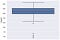Diagram shows a basic box plot that shows the height of a group of people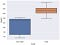Diagram shows a basic box plot for multiple variables. In this case, it shows heights for two different groups — adults and non-adults

# 4. Violin Plot

Violin plots are a combination of box plots and density plots. Both the statistical measures and the distribution are visualized. The thick black bar in the center represents the interquartile range, while the thin black line corresponds to the whiskers in a box plot. The white dot indicates the median. On both sides of the center line, the density is visualized.

Compare statistical measures and density for multiple variables or groups.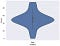Diagram shows a violin plot for a single variable and shows how students have performed in Math

From the preceding diagram, we can analyze that most of the students have scored around 40–60 in the Math test.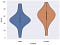Diagram shows a violin plot for two variables and shows the performance of students in English and Math

From the preceding diagram, we can say that on average, the students have scored more in English than in Math, but the highest score was secured in Math.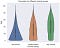Diagram shows a violin plot for a single variable divided into three groups, and shows the performance of three divisions of students in English based on their score

From the preceding diagram, we can note that on average, division C has scored the highest, division B has scored the lowest, and division A is, on average, in between divisions B and C.

Design Practice

• Scale the axes accordingly so that the distribution is clearly visible and not flat.

In this article, distribution plots were introduced and I hope you learned a lot from it. In the later article, we will have a closer look at histograms.

For more awesome content and regular posts you can connect with me on Instagram😍

CEO Techneophyte | Python Developer | ML Engineer | Data Scientist | Flutter Developer | Penetration Tester | Software Engineer at Infosys

## More from Abhijeet Srivastav

CEO Techneophyte | Python Developer | ML Engineer | Data Scientist | Flutter Developer | Penetration Tester | Software Engineer at Infosys